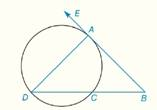Chapter 6.CR, Problem 9CR### Elementary Geometry for College St...

6th Edition
Daniel C. Alexander + 1 other
ISBN: 9781285195698

#### Solutions

Chapter
Section### Elementary Geometry for College St...

6th Edition
Daniel C. Alexander + 1 other
ISBN: 9781285195698
Textbook Problem
1 views

# In Review Exercises 5 to 10, B A → is tangent to the circle at point A in the figure shown. G i v e n :           C   i s   t h e   m i d p o i n t   o f   A C D ^   a n d     m ∠ B = 40 °       F i n d :             m A D ^ ,   m A C ^ , m D C ^To determine

Explanation

Theorem:

1) The measure of an angle formed when two secants intersect at a point outside the circle is one-half the difference of the measures of two intercepted arcs.

2) The measure of an inscribed angle of a circle is one-half the measure of its intercepted arc.

Calculation:

Given that

mB=40° and C is the midpoint of mACD^ and BA is tangent to the circle at point A.

By theorem, the measure of an angle (mB) formed when two secants (BD and BA) intersect at a point (B) outside the circle is one-half the difference of the measures of two intercepted arcs AD^ and AC^ .

Since, C is the midpoint of mACD^, mAC^=mDC^

### Still sussing out bartleby?

Check out a sample textbook solution.

See a sample solution

#### The Solution to Your Study Problems

Bartleby provides explanations to thousands of textbook problems written by our experts, many with advanced degrees!

Get Started

#### Find more solutions based on key concepts